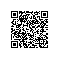# 记录遇到的Python陷阱和注意点

## 避免可变对象作为默认参数

def append_item(a = 1, b = []):
b.append(a)
print b

append_item(a=1)
append_item(a=3)
append_item(a=5)  


[1, 3]
[1, 3, 5]

class Test(object):
def __init__(self):
print("Init Test")

def arg_init(a, b = Test()):
print(a)

arg_init(1)
arg_init(3)
arg_init(5)

Init Test
1
3
5

### 可变默认参数的正确使用

def append_item(a = 1, b = None):
if b is None:
b = []
b.append(a)
print b

append_item(a=1)
append_item(a=3)
append_item(a=5) 





## Python中的作用域

Python的作用域解析顺序为Local、Enclosing、Global、Built-in，也就是说Python解释器会根据这个顺序解析变量。

global_var = 0

def outer_func():
outer_var = 1

def inner_func():
inner_var = 2

print "global_var is :", global_var
print "outer_var is :", outer_var
print "inner_var is :", inner_var

inner_func()

outer_func()

global_var is : 0
outer_var is : 1
inner_var is : 2

num = 0

def var_func():
num = 1
print "num is :", num

var_func()

### 问题一

num = 0

def var_func():
print "num is :", num
num = 1

var_func()

UnboundLocalError: local variable 'num' referenced before assignment

### 问题二

li = [1, 2, 3]

def foo():
li.append(4)
print li

foo()

def bar():
li +=
print li

bar()

[1, 2, 3, 4]
UnboundLocalError: local variable 'li' referenced before assignment

foo函数中，根据Python的作用域解析顺序，该函数中使用了全局的li变量；但是在bar函数中，对li变量进行了赋值，所以li会被当作bar作用域中的变量。

li = [1, 2, 3]

def foo():
li.append(4)
print li

foo()

def bar():
global li
li +=
print li

bar()

## 类属性隐藏

class Student(object):
books = ["Python", "JavaScript", "CSS"]
def __init__(self, name, age):
self.name = name
self.age = age
pass

wilber = Student("Wilber", 27)
print "%s is %d years old" %(wilber.name, wilber.age)

print Student.books
print wilber.books

wilber.books = ["HTML", "AngularJS"]

print Student.books
print wilber.books

del wilber.books

print Student.books
print wilber.books

Wilber is 27 years old
['Python', 'JavaScript', 'CSS']
['Python', 'JavaScript', 'CSS']
['Python', 'JavaScript', 'CSS']
['HTML', 'AngularJS']
['Python', 'JavaScript', 'CSS']
['Python', 'JavaScript', 'CSS']

class A(object):
count = 1
class B(A):
pass
class C(A):
pass
print A.count, B.count, C.count
B.count = 2
print A.count, B.count, C.count
A.count = 3
print A.count, B.count, C.count
print B.__dict__
print C.__dict__

1 1 1
1 2 1
3 2 3
{'count': 2, '__module__': '__main__', '__doc__': None}
{'__module__': '__main__', '__doc__': None}

但是，仍然存在一些不是很直观的地方，特别是对C++和Java程序员来说，更是如此。

在这里，我们需要明白以下几个地方：

1.Python是一门动态语言，任何实体都可以动态地添加或删除属性。

2.一个类定义了一个作用域。

3.类实例也引入了一个作用域，这与相应类定义的作用域不同。

4.在类实例中查找属性的时候，首先在实例自己的作用域中查找，如果没有找到，则再在类定义的作用域中查找。

5.在对类实例属性进行赋值的时候，实际上会在类实例定义的作用域中添加一个属性（如果还不存在的话），并不会影响到相应类中定义的同名属性。

下面看一个例子，加深对上述几点的理解：

class A:
cls_i = 0
cls_j = {}
def __init__(self):
self.instance_i = 0
self.instance_j = {}

>>> a = A()
>>> a.__dict__
{'instance_j': {}, 'instance_i': 0}
>>> A.__dict__
{'__init__': , '__module__': '__main__', 'cls_i': 0, 'cls_j': {}, '__doc__': None}


我们再来看看名字查找是如何发生的：

>>> a.cls_i
0
>>> a.instance_i
0
在查找cls_i的时候，实例a的作用域中是没有它的，却在A的作用域中找到了它；在查找instance_i的时候，直接可在a的作用域中找到它。

如果我们企图通过实例a来修改cls_i的值，那会怎样呢：
>>> a.cls_i = 1
>>> a.__dict__
{'instance_j': {}, 'cls_i': 1, 'instance_i': 0}
>>> A.__dict__
{'__init__': , '__module__': '__main__', 'cls_i': 0, 'cls_j': {}, '__doc__': None}

如果我们通过实例a操纵cls_j中的数据（注意不是cls_j本身），又会怎么样呢：

>>> a.cls_j['a'] = 'a'
>>> a.__dict__
{'instance_j': {}, 'cls_i': 1, 'instance_i': 0}
>>> A.__dict__
{'__init__': , '__module__': '__main__', 'cls_i': 0, 'cls_j': {'a': 'a'}, '__doc__': None}

实例的作用域发生变化，并不会影响到该类的其它实例，但是类的作用域发生变化，则会影响到该类的所有实例，包括在这之前创建的实例：

>>> A.cls_k = 0
>>> i.cls_k
0
http://www.cnblogs.com/frydsh/p/3194710.html

## tuple是“可变的”

tpl = (1, 2, 3, [4, 5, 6])
print id(tpl)
print id(tpl)
tpl.extend([7, 8])
print tpl
print id(tpl)
print id(tpl)

36764576
38639896
(1, 2, 3, [4, 5, 6, 7, 8])
36764576
38639896

## Python的深浅拷贝

• 使用切片[:]操作
• 使用工厂函数（如list/dir/set）
• 使用copy模块中的copy()函数

import copy

will = ["Will", 28, ["Python", "C#", "JavaScript"]]
wilber = copy.copy(will)

print id(will)
print will
print [id(ele) for ele in will]
print id(wilber)
print wilber
print [id(ele) for ele in wilber]

will = "Wilber"
will.append("CSS")
print id(will)
print will
print [id(ele) for ele in will]
print id(wilber)
print wilber
print [id(ele) for ele in wilber]

import copy

will = ["Will", 28, ["Python", "C#", "JavaScript"]]
wilber = copy.deepcopy(will)

print id(will)
print will
print [id(ele) for ele in will]
print id(wilber)
print wilber
print [id(ele) for ele in wilber]

will = "Wilber"
will.append("CSS")
print id(will)
print will
print [id(ele) for ele in will]
print id(wilber)
print wilber
print [id(ele) for ele in wilber]

## 模块循环依赖

# module_x.py
import module_y

def inc_count():
module_y.count += 1
print module_y.count

# module_y.py
import module_x

count = 10

def run():
module_x.inc_count()

run()            

# module_x.py
def inc_count():
import module_y
module_y.count += 1
print module_y.count使用钉钉扫一扫加入圈子
+ 订阅